• Save

Logarithm problems questions and answers for SSC and Bank exams

4 months ago 10.3K Views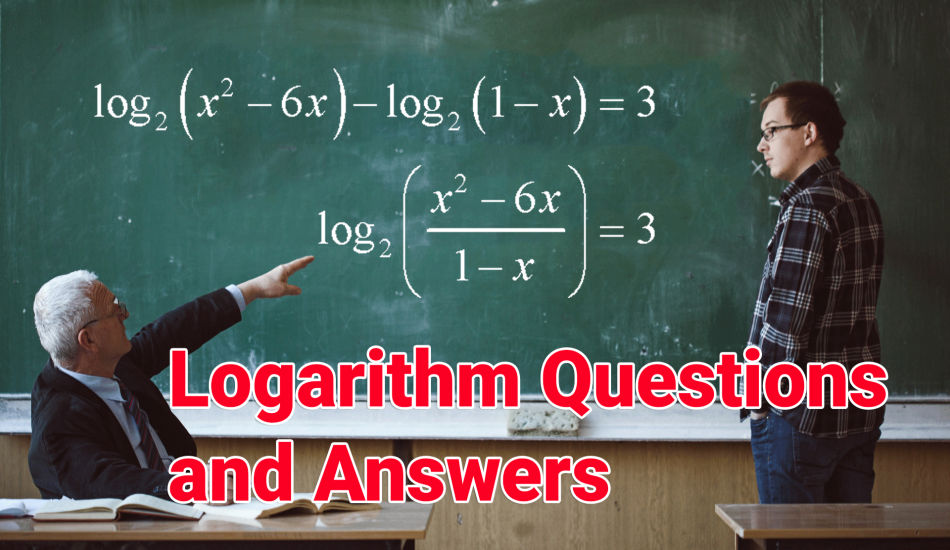Logarithm problems have been asked in many competitive exams and there are strong chances to ask Logarithm aptitude questions again in SSC, Bank and other competitive exams. Students should prepare for exams by practicing Logarithm questions and answers.

Logarithm questions are important and 1-2 questions asked in exams every year, try to solve Logarithm problems and logarithm questions and answers for better results in upcoming competitive exams.

Let's find some important logarithm equations and examples with solutions for SSC and Bank exams for self-practice. You should understand also how to use formula and equations with examples in logarithm questions for save your time in competitive exams.

Logarithm questions and answers for Competitive exams:

1.The value of log343 7 is:

(A)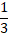(B) 3

(C) – 3

(D) )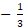Ans .   A

2. The value of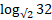is:

(A)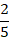(B)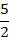(C) 10

(D)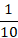Ans .   C

3. The value of log (0.1) (1000) is:

(A)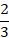(B)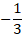(C)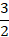(D) 3

Ans .   B

4. If log10000 x =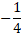, then x is equal to:

(A)(B)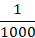(C)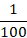(D)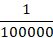Ans .   A

5. If logx (0.1) =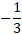, then x is equal to:

(A)(B) 10

(C) 1000

(D)Ans .   C

6. The value of log2 (log5 625) is:

(A) 2

(B) 7

(C) 14

(D) 15

Ans .   A

7. The value of log2 log2 loglog3 273 is:

(A) 0

(B) 2

(C) 64

(D) 512

Ans .   A

8. log 360 is equal to:

(A) 3 log 2 + 2 log 3

(B) 2 log 2 + 3 log 3

(C) 3 log 2 + 2 log 3 – log 5

(D) 3 log 2 + 2 log 3 + log 5

Ans .   D

If you want to read more questions and answers of logarithm problems, visit the next page. if you face any problem or you have any query related logarithm problems, you can ask me in the comment section.